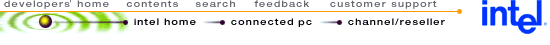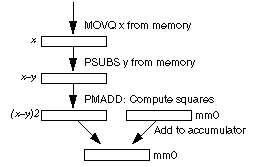## Using MMX™ Instructions to Compute the L2 Norm Between Two 16-Bit Vectors

 Disclaimer Information in this document is provided in connection with Intel products. No license, express or implied, by estoppel or otherwise, to any intellectual property rights is granted by this document. Except as provided in Intel's Terms and Conditions of Sale for such products, Intel assumes no liability whatsoever, and Intel disclaims any express or implied warranty, relating to sale and/or use of Intel products including liability or warranties relating to fitness for a particular purpose, merchantability, or infringement of any patent, copyright or other intellectual property right. Intel products are not intended for use in medical, life saving, or life sustaining applications. Intel may make changes to specifications and product descriptions at any time, without notice. Copyright © Intel Corporation (1996). Third-party brands and names are the property of their respective owners.

 1.0. INTRODUCTION 2.1. Core of the L2 Norm 2.2. Scheduling for Optimum Pairing

## 1.0 INTRODUCTION

The Intel Architecture (IA) media extensions include single-instruction, multi-data (SIMD) instructions. This application note presents a code example that uses these MMX instructions to implement a 16-bit L2 Norm function.

## 2.0. THE L2 NORM FUNCTION

The L2 Norm function, also called the Euclidean distance, takes two vectors x and y and computes:

L2Norm = S ( xi - yi )2

In this application note, the input vectors x and y are assumed to be arrays of 16-bit words and the sum is 32 bits.

The core of an L2 Norm computation takes four MMX instructions, as shown in Figure 1. This sequence of four instructions adds the contributions of four terms to the partial sum.

The first instruction, MOVQ, loads four consecutive elements of the x array into the MM1 register. The next instruction, PSUBS, calculates xi - yi, with the y values coming from memory. The third instruction does a PMADD MM1,MM1, which calculates the square of all four 16-bit SIMD fields and adds them together in pairs. The final instruction is a PADDD which adds the two 32-bit results in MM1 to an accumulator register (MM0).

Figure 1. Core of the L2 Norm AlgorithmIn order to get the best performance, the code must be properly interleaved to avoid read-after-write dependencies and to satisfy the other pairing and latency rules of the Pentium® processor.

The following four instructions are the core of the algorithm:

• L: Load x from memory (MOVQ)

• S: Subtract y from memory (PSUBS)

For optimum performance, the instructions for one iteration need to satisfy the following rules:

• The S must be one clock after the L instruction. In other words, both instructions cannot be in the same clock (one in the U pipe and one in the V pipe) since the S instruction uses the result of the L instruction.

• Similarly, the M instruction must be one clock after the S instruction, since it uses the result of the S instruction.

• The A instruction must be three clocks after the M instruction, because there is a latency of three clocks in the MMX technology multiplier before the result is ready.

• It is not possible to pair an L instruction and an S instruction (even from different iterations), because they both access memory and only one memory access is allowed per clock.

It is possible to interleave four copies of the basic sequence (representing iterations n, n+1, n+2, n+3 of the algorithm) and satisfy the optimization rules, thereby achieving perfect U, V pairing and optimum performance. This sequence is shown schematically in Example 1.

To this basic sequence it is necessary to add at least two instructions for loop control-one to decrement an index register and a conditional jump. If these two instructions are placed at the end, they will pair and only take one clock; however, there will be a one-clock penalty at the top of the loop, since the index register is used to reference memory.

In order to eliminate this penalty, we can take advantage of the fact that you can pair an MMX instruction and a pairable integer instruction. This permits the loop control to be combined with the last two instructions in Example 1. The index decrement can be paired with the last S, and the conditional jump can be paired with the last A.

The final form of the loop is shown in the complete code listing in Section 4. Note that when the loop finishes, the 3rd and 4th iteration (i.e., the columns labeled n+2 and n+3 in Example 1) need to be "finished off," by doing the A for n+2 and the M and A for n+3. This is accomplished by the three instructions after the bottom of the loop in the code listing.

## 3.0. PERFORMANCE

This section describes the performance of the MMX technology L2 Norm and compares it to an implementation using the scalar Intel Architecture instructions.

Example 1. L2 Norm with U, V Pairing
```			Iter:	n	n+1	n+2	n+3

U	L
V				M
U	S
V			A
etc..		L
M
S
A
L
M
S
A
L
M
S
A

```

The loop shown in Example 1 computes 16 terms of the L2 Norm, since each basic sequence (L,S,M,A) computes four terms and four sequences have been interleaved. The instructions in Example 1 take eight clocks, and one additional clock is required for loop control (as discussed in Section 2.2), for a total of nine clocks. Therefore, the speed of the MMX technology L2 Norm is:

MMX Technology L2 Norm = 9/16 = 0.5625 clocks per term

To compute an L2 Norm on a Pentium processor without using MMX instructions, the most efficient method would be to recast the algorithm so that the data is in floating-point, and use floating-point instructions. In this case, four floating-point instructions (FLD, FSUB, FMUL, FADD) are required per term of the L2 Norm, plus two clocks for loop control. If the loop is unrolled 16 times, this means that the performance of a floating-point L2 Norm is:

Scalar L2 Norm = 66/16 = 4.125 clocks per term

The MMX technology implementation of the 16-bit L2 Norm is therefore about 7.3 times faster than a floating-point version. If compared against an integer scalar implementation, the difference is even greater (about a factor of 20) due to the number of clocks consumed by the IMUL instruction.

## 4.0. CODE LISTING: L2 Norm

```
.486p
.Model flat, c
.code

; L2 norm on 16-bit vectors using MMX code.  For simplicity, this
; routine REQUIRES the arrays to be a multiple of 16 (words) in size.
; This is because one iteration handles 4 words, and we have interleaved
; four iterations for maximum utilization of the U,V pipes.
; Handling arbitrary length is left as an exercise for the reader.

l2norm PROC C PUBLIC USES esi edi ebx \
in1:DWORD, in2:DWORD, len:DWORD

; in1,in2 are word arrays
; Len is number of words

mov     esi,in1
mov     ecx,len
mov     edi,in2
shr     ecx,2
dec     ecx
pxor    mm0,mm0    ; set accumulator to zero
pxor    mm3,mm3    ; mm3=0 so first A in col 3 has no effect
pxor    mm4,mm4    ; mm4=0 so first M and A in
; col 4 have no effect

loop1:
;  1  2  3  4

movq mm1,[esi+8*ecx]                               ;  L

psubsw mm1,[edi+8*ecx]                             ;  S

movq mm2,[esi+8*ecx-8]                             ;  L

psubsw mm2,[edi+8*ecx-8]                           ;  S

movq mm3,[esi+8*ecx-16]                            ;  L

psubsw mm3,[edi+8*ecx-16]                          ;  S

movq mm4,[esi+8*ecx-24]                            ;  L

psubsw mm4,[edi+8*ecx-24]                          ;  S
sub     ecx,4
jge     loop1

; finish up columns 3 (need an A) and 4 (need M and A)

; add the two dwords in the accumulator together

movq    mm1,mm0
psrlq   mm1,32